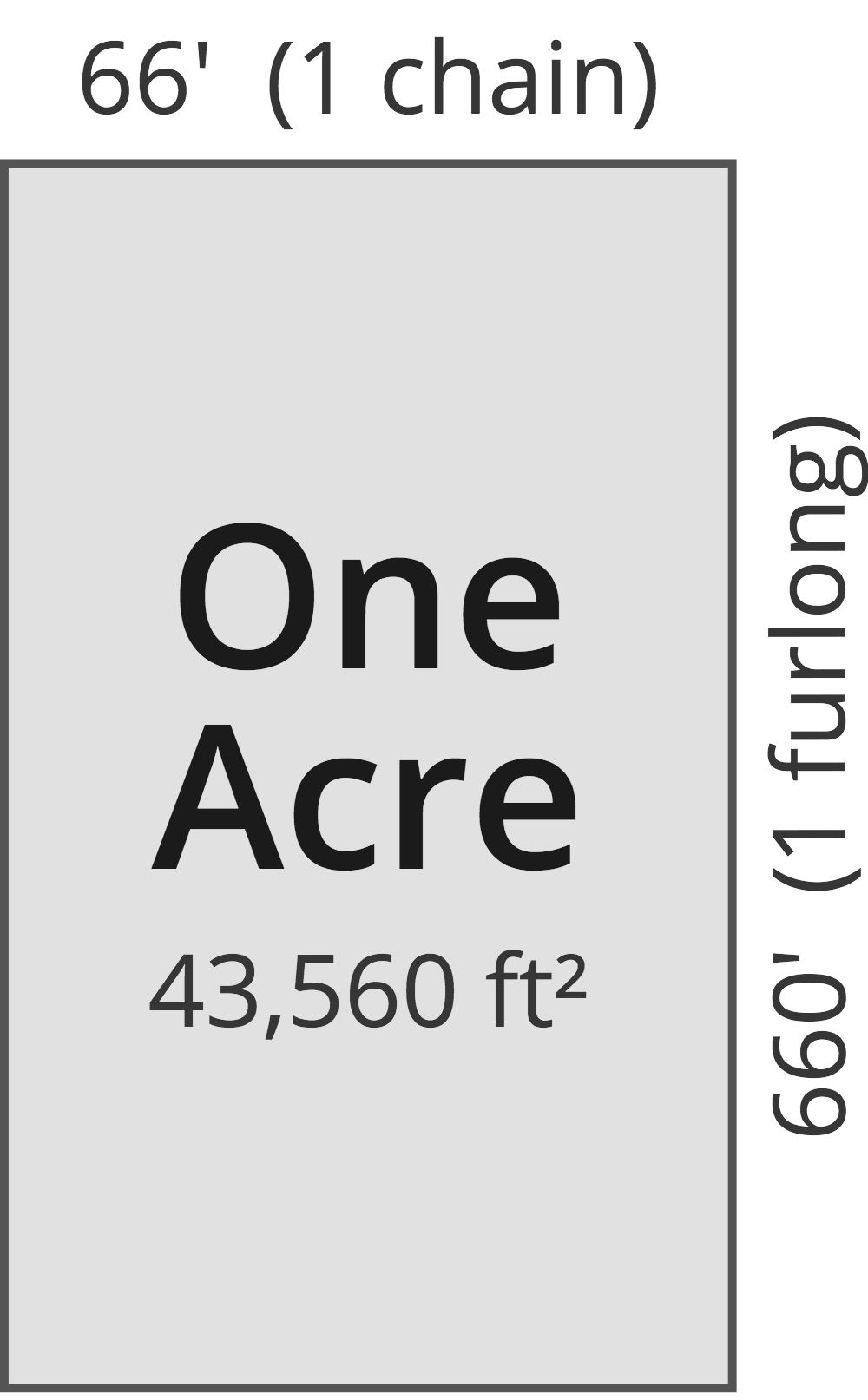# Square Millimeters to Acres Converter

Enter the area in square millimeters below to get the value converted to acres.

Results in Acres:1 sq mm = 2.4711E-10 ac
Hint: use a scientific notation calculator to convert E notation to decimal

Do you want to convert acres to square millimeters?

## How to Convert Square Millimeters to Acres

To convert a measurement in square millimeters to a measurement in acres, multiply the area by the following conversion ratio: 2.4711E-10 acres/square millimeter.

Since one square millimeter is equal to 2.4711E-10 acres, you can use this simple formula to convert:

acres = square millimeters × 2.4711E-10

The area in acres is equal to the area in square millimeters multiplied by 2.4711E-10.

For example, here's how to convert 5,000,000,000 square millimeters to acres using the formula above.
acres = (5,000,000,000 sq mm × 2.4711E-10) = 1.235527 ac

## What Is a Square Millimeter?

One square millimeter is equivalent to the area of a square with edges that are each 1 millimeter in length. One square millimeter is equal to 0.00155 square inches or 1/100 of a square centimeter.

The square millimeter, or square millimetre, is a multiple of the square meter, which is the SI derived unit for area. In the metric system, "milli" is the prefix for thousandths, or 10-3. A square millimeter is sometimes also referred to as a square mm. Square millimeters can be abbreviated as sq mm, and are also sometimes abbreviated as mm². For example, 1 square millimeter can be written as 1 sq mm or 1 mm².

## What Is an Acre?

One acre is defined as the area equal to a space that is one chain (66 ft) by one furlong (660 ft), or 10 square chains. That's equal to 43,560 square feet or 1/640 of a square mile for those unfamiliar with those units of measure.The acre is a US customary and imperial unit of area. Acres can be abbreviated as ac; for example, 1 acre can be written as 1 ac.

You can use an acreage calculator to measure the area of a plot of land in acres by locating the boundaries on a map.

## Square Millimeter to Acre Conversion Table

Table showing various square millimeter measurements converted to acres.
Square Millimeters Acres
1 sq mm 0.00000000024711 ac
2 sq mm 0.00000000049421 ac
3 sq mm 0.00000000074132 ac
4 sq mm 0.00000000098842 ac
5 sq mm 0.0000000012355 ac
6 sq mm 0.0000000014826 ac
7 sq mm 0.0000000017297 ac
8 sq mm 0.0000000019768 ac
9 sq mm 0.0000000022239 ac
10 sq mm 0.0000000024711 ac
100 sq mm 0.000000024711 ac
1,000 sq mm 0.00000024711 ac
10,000 sq mm 0.0000024711 ac
100,000 sq mm 0.000024711 ac
1,000,000 sq mm 0.000247 ac
10,000,000 sq mm 0.002471 ac
100,000,000 sq mm 0.024711 ac
1,000,000,000 sq mm 0.247105 ac
10,000,000,000 sq mm 2.4711 ac

## References

1. National Institute of Standards and Technology, Specifications, Tolerances, and Other Technical Requirements for Weighing and Measuring Devices, Handbook 44 - 2019 Edition, https://nvlpubs.nist.gov/nistpubs/hb/2019/NIST.HB.44-2019.pdf